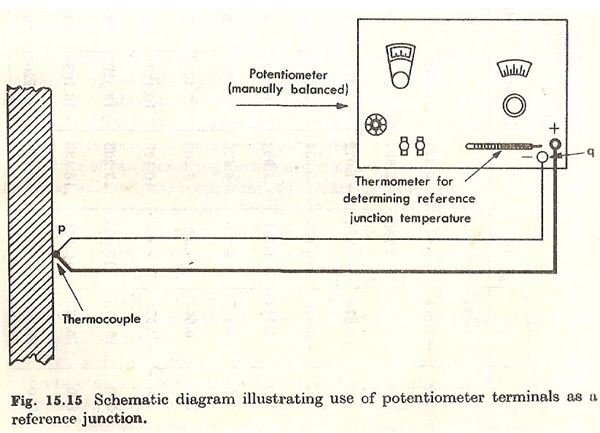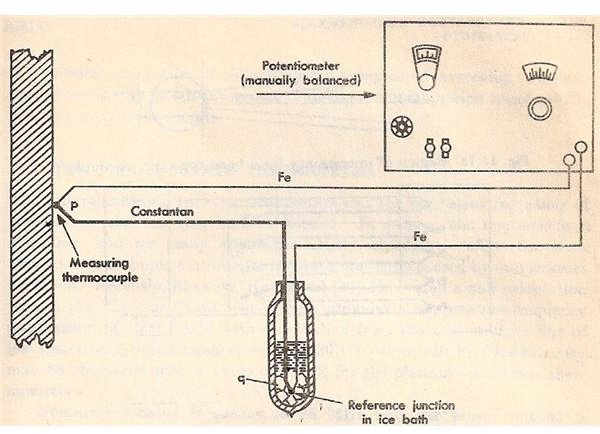# What is a Thermocouple & How Does it Work? Principle of Working of Thermocouple

Page content

A thermocouple is comprised of at least two metals joined together to form two junctions. One is connected to the body whose temperature is to be measured; this is the hot or measuring junction. The other junction is connected to a body of known temperature; this is the cold or reference junction. Therefore the thermocouple measures unknown temperature of the body with reference to the known temperature of the other body.

## Working Principle

The working principle of thermocouple is based on three effects, discovered by Seebeck, Peltier and Thomson. They are as follows:

1) Seebeck effect: The Seebeck effect states that when two different or unlike metals are joined together at two junctions, an electromotive force (emf) is generated at the two junctions. The amount of emf generated is different for different combinations of the metals.

2) Peltier effect: As per the Peltier effect, when two dissimilar metals are joined together to form two junctions, emf is generated within the circuit due to the different temperatures of the two junctions of the circuit.

3) Thomson effect: As per the Thomson effect, when two unlike metals are joined together forming two junctions, the potential exists within the circuit due to temperature gradient along the entire length of the conductors within the circuit.

In most of the cases the emf suggested by the Thomson effect is very small and it can be neglected by making proper selection of the metals. The Peltier effect plays a prominent role in the working principle of the thermocouple.

## Diagrams## How it Works

The general circuit for the working of thermocouple is shown in the figure 1 above. It comprises of two dissimilar metals, A and B. These are joined together to form two junctions, p and q, which are maintained at the temperatures T1 and T2 respectively. Remember that the thermocouple cannot be formed if there are not two junctions. Since the two junctions are maintained at different temperatures the Peltier emf is generated within the circuit and it is the function of the temperatures of two junctions.

If the temperature of both the junctions is same, equal and opposite emf will be generated at both junctions and the net current flowing through the junction is zero. If the junctions are maintained at different temperatures, the emf’s will not become zero and there will be a net current flowing through the circuit. The total emf flowing through this circuit depends on the metals used within the circuit as well as the temperature of the two junctions. The total emf or the current flowing through the circuit can be measured easily by the suitable device.

The device for measuring the current or emf is connected within the circuit of the thermocouple. It measures the amount of emf flowing through the circuit due to the two junctions of the two dissimilar metals maintained at different temperatures. In figure 2 the two junctions of the thermocouple and the device used for measurement of emf (potentiometer) are shown.

Now, the temperature of the reference junctions is already known, while the temperature of measuring junction is unknown. The output obtained from the thermocouple circuit is calibrated directly against the unknown temperature. Thus the voltage or current output obtained from thermocouple circuit gives the value of unknown temperature directly.

## Devices Used for Measuring EMF

The amount of emf developed within the thermocouple circuit is very small, usually in millivolts, therefore highly sensitive instruments should be used for measuring the emf generated in the thermocouple circuit. Two devices used commonly are the ordinary galvanometer and voltage balancing potentiometer. Of those two, a manually or automatically balancing potentiometer is used most often.

Figure 2 shows the potentiometer connected in the thermocouple circuit. The junction p is connected to the body whose temperature is to be measured. The junction q is the reference junction, whose temperature can be measured by the thermometer. In some cases the reference junctions can also be maintained at the ice temperature by connecting it to the ice bath (see figure 3). This device can be calibrated in terms of the input temperature so that its scale can give the value directly in terms of temperature.

## Reference

Book: Mechanical Measurements by Thomas G. Beckwith and N. Lewis Buck

## Images Courtesy

1. Book: Mechanical Measurements by Thomas G. Beckwith and N. Lewis Buck

## This post is part of the series: What are Thermocouples? How Thermocouples Work?

This is the series of articles that describes what are thermocouples, how thermocouples work, materials used for thermocouples, and various shapes and forms of thermocouples.